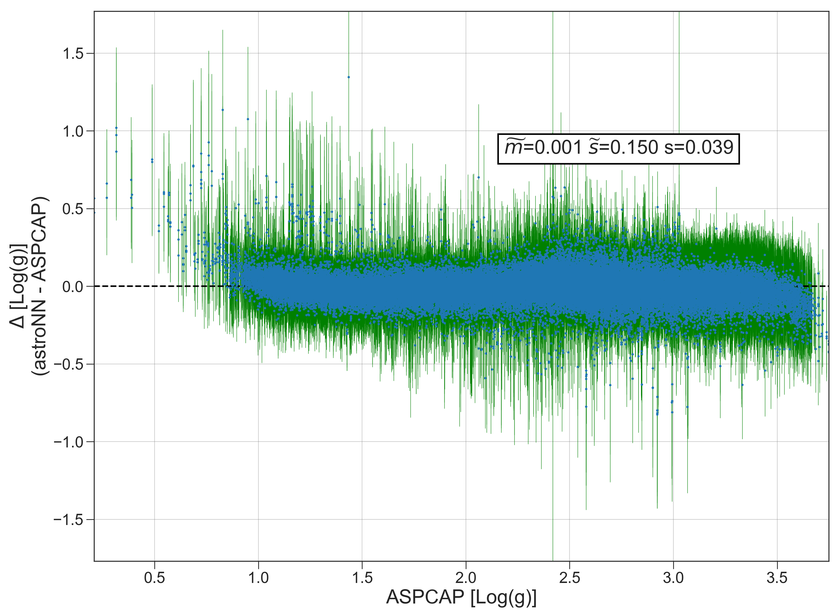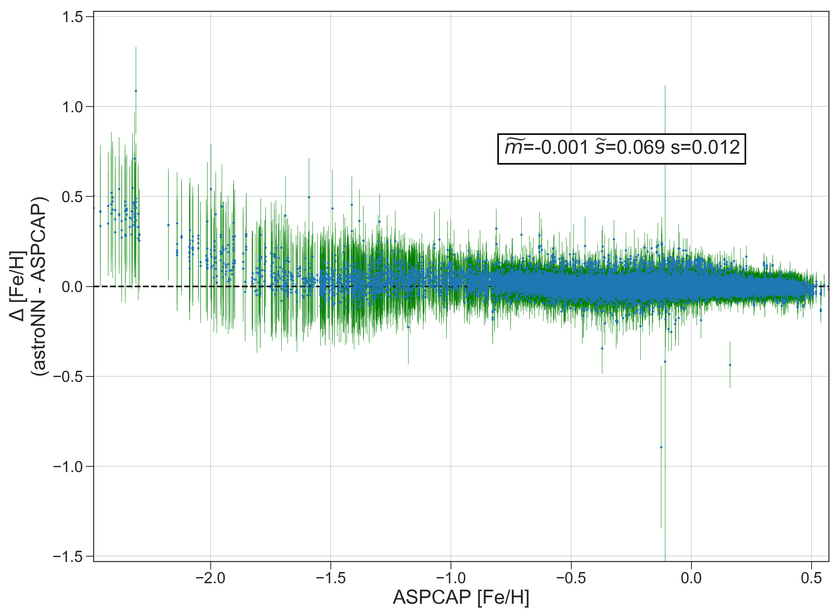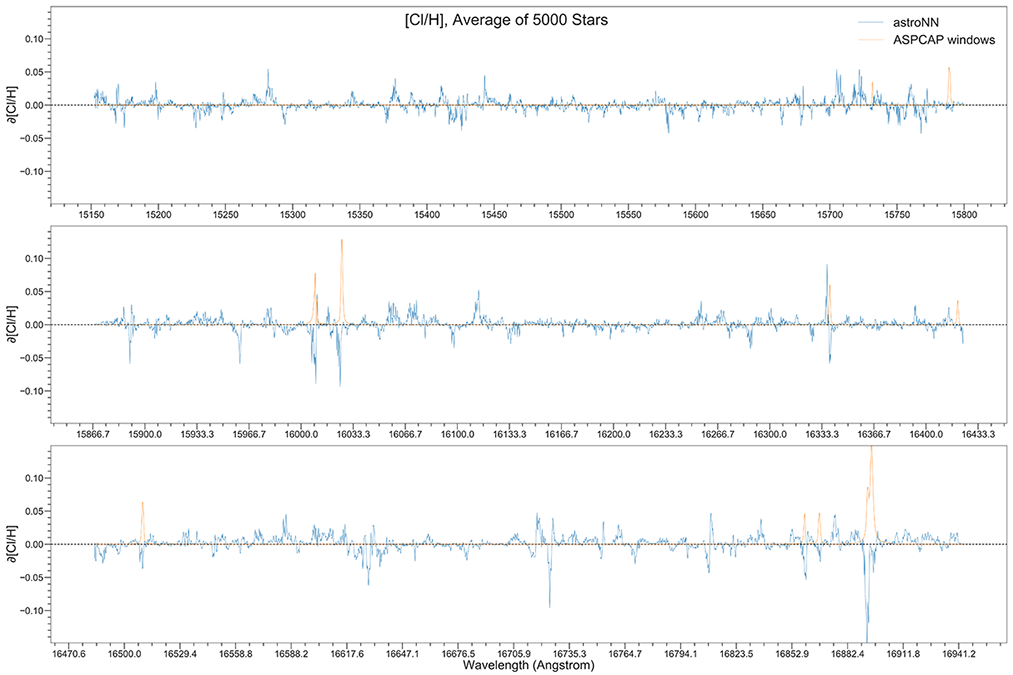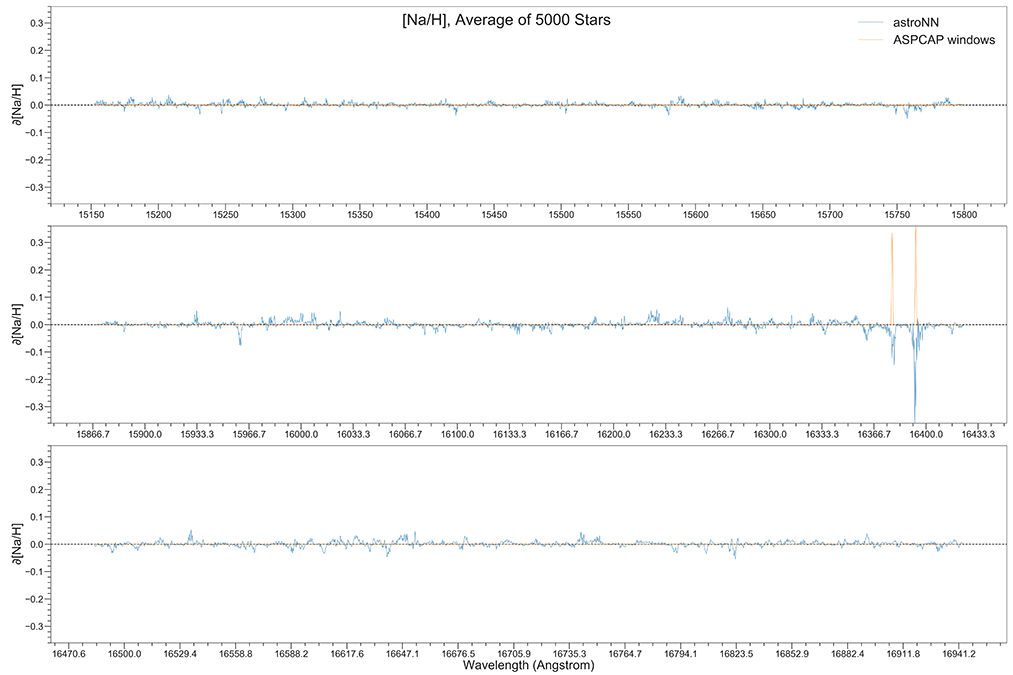# APOGEE Spectra with Bayesian Neural Net - astroNN.models.ApogeeBCNN

class astroNN.models.apogee_models.ApogeeBCNN(lr=0.0005, dropout_rate=0.3)[source]

Class for Bayesian convolutional neural network for stellar spectra analysis

History

2017-Dec-21 - Written - Henry Leung (University of Toronto)Although in theory you can feed any 1D data to astroNN neural networks. This tutorial will only focus on spectra analysis.

```from astroNN.models import ApogeeBCNN

# Load the train data from dataset first, x_train is spectra and y_train will be ASPCAP labels

# And then create an instance of Bayesian Convolutional Neural Network class
bcnn_net = ApogeeBCNN()

# You don't have to specify the task because its 'regression' by default. But if you are doing classification. you can set task='classification'

# Set max_epochs to 10 for a quick result. You should train more epochs normally, especially with dropout
bcnn_net.max_epochs = 10
bcnn_net.train(x_train, y_train, x_err, y_err)
```

Here is a list of parameter you can set but you can also not set them to use default

```ApogeeBCNN.batch_size = 64
ApogeeBCNN.initializer = 'he_normal'
ApogeeBCNN.activation = 'relu'
ApogeeBCNN.num_filters = [2, 4]
ApogeeBCNN.filter_len = 8
ApogeeBCNN.pool_length = 4
ApogeeBCNN.num_hidden = [196, 96]
ApogeeBCNN.max_epochs = 100
ApogeeBCNN.lr = 0.005
ApogeeBCNN.reduce_lr_epsilon = 0.00005
ApogeeBCNN.reduce_lr_min = 0.0000000001
ApogeeBCNN.reduce_lr_patience = 10
ApogeeBCNN.target = 'all'
ApogeeBCNN.l2 = 5e-9
ApogeeBCNN.dropout_rate = 0.2
ApogeeBCNN.length_scale = 0.1  # prior length scale
ApogeeBCNN.input_norm_mode = 3
ApogeeBCNN.labels_norm_mode = 2
```

Note

You can disable astroNN data normalization via `ApogeeBCNN.input_norm_mode=0` as well as `ApogeeBCNN.labels_norm_mode=0` and do normalization yourself. But make sure you don’t normalize labels with MAGIC_NUMBER (missing labels).

After the training, you can use bcnn_net in this case and call test method to test the neural network on test data. Or you can load the folder by

```from astroNN.models import load_folder

# Load the test data from dataset, x_test is spectra and y_test will be ASPCAP labels

# pred contains denormalized result aka. ASPCAP labels prediction in this case
# pred_std is a list of uncertainty
# pred_std['total'] is the total uncertainty (standard derivation) which is the sum of all the uncertainty
# pred_std['predictive'] is the predictive uncertainty predicted by bayesian neural net
# pred_std['model'] is the model uncertainty from dropout variational inference
pred, pred_std = bcnn_net.test(x_test)
```

Since astroNN.models.ApogeeBCNN uses Bayesian deep learning which provides uncertainty analysis features.

You can calculate jacobian which represents the output derivative to the input and see where those output is sensitive to in inputs.

```# Calculate jacobian first
jacobian_array = bcnn_net.jacobian(x_test, mean_output=True)
```

Note

You can access to Keras model method like model.predict via (in the above tutorial) bcnn_net.keras_model (Example: bcnn_net.keras_model.predict())

## ASPCAP Labels Prediction

Internal model identifier for the author: `astroNN_0321_run002`

Training set (30067 spectra + separate 3340 validation spectra): Starflag=0 and ASPCAPflag=0, 4000<Teff<5500, 200<SNR

Testing set (97723 spectra): Individual Visit of the training spectra, median SNR is around SNR~100

Using astroNN.models.ApogeeBCNN with default hyperparameter

Ground Truth is ASPCAP labels.

Median of residue

Al

-0.003

0.042

Alpha

0.000

0.013

C

0.003

0.032

C1

0.005

0.037

Ca

0.002

0.022

Co

-0.005

0.071

Cr

-0.001

0.031

fakemag

3.314

16.727

Fe

0.001

0.016

K

-0.001

0.032

Log(g)

0.002

0.048

M

0.003

0.015

Mg

0.001

0.021

Mn

0.003

0.025

N

-0.002

0.037

Na

-0.006

0.103

Ni

0.000

0.021

O

0.004

0.027

P

0.005

0.086

S

0.006

0.043

Si

0.001

0.022

Teff

0.841

23.574

Ti

0.002

0.032

Ti2

-0.009

0.089

V

-0.002

0.059

Median Absolute Error of prediction at three different low SNR level.

SNR ~ 20

SNR ~ 40

SNR ~ 60

Al

0.122 dex

0.069 dex

0.046 dex

Alpha

0.024 dex

0.017 dex

0.014 dex

C

0.088 dex

0.051 dex

0.037 dex

C1

0.084 dex

0.054 dex

0.041 dex

Ca

0.069 dex

0.039 dex

0.029 dex

Co

0.132 dex

0.104 dex

0.085 dex

Cr

0.082 dex

0.049 dex

0.037 dex

fakemag

Not Calculated

Not Calculated

Not Calculated

Fe

0.070 dex

0.035 dex

0.024 dex

K

0.091 dex

0.050 dex

0.037 dex

Log(g)

0.152 dex

0.085 dex

0.059 dex

M

0.067 dex

0.033 dex

0.023 dex

Mg

0.080 dex

0.039 dex

0.026 dex

Mn

0.089 dex

0.050 dex

0.037 dex

N

0.118 dex

0.067 dex

0.046 dex

Na

0.119 dex

0.110 dex

0.099 dex

Ni

0.076 dex

0.039 dex

0.027 dex

O

0.076 dex

0.046 dex

0.037 dex

P

0.106 dex

0.082 dex

0.077 dex

S

0.072 dex

0.052 dex

0.041 dex

Si

0.076 dex

0.042 dex

0.024 dex

Teff

74.542 K

41.955 K

29.271 K

Ti

0.080 dex

0.049 dex

0.037 dex

Ti2

0.124 dex

0.099 dex

0.092 dex

V

0.119 dex

0.080 dex

0.064 dex

## ASPCAP Labels Prediction with >50% corrupted labels

Internal model identifier for the author: `astroNN_0224_run004`

Setting is the same as above, but manually corrupt more labels to ensure the modified loss function is working fine

52.5% of the total training labels is corrupted to -9999 (4.6% of the total labels are -9999. from ASPCAP), while testing set is unchanged

Median of residue

Al

0.003

0.047

Alpha

0.000

0.015

C

0.005

0.037

C1

0.003

0.042

Ca

0.002

0.025

Co

0.001

0.076

Cr

0.000

0.033

fakemag

-0.020

5.766

Fe

0.001

0.020

K

0.001

0.035

Log(g)

-0.002

0.064

M

0.002

0.019

Mg

0.003

0.025

Mn

0.003

0.030

N

0.001

0.043

Na

-0.004

0.106

Ni

0.001

0.025

O

0.004

0.031

P

0.004

0.091

S

0.006

0.045

Si

0.001

0.026

Teff

-0.405

31.222

Ti

0.003

0.035

Ti2

-0.012

0.092

V

0.002

0.063

## ASPCAP Labels Prediction with limited amount of data

Internal model identifier for the author: `astroNN_0401_run001`

Setting is the same including the neural network, but the number of training data is limited to 5000 (4500 of them is for training, 500 validation), validation set is completely separated. Testing set is the same without any limitation.

Median of residue

Al

-0.002

0.051

Alpha

0.001

0.017

C

-0.002

0.040

C1

-0.003

0.046

Ca

-0.003

0.027

Co

-0.006

0.080

Cr

0.000

0.036

fakemag

18.798

30.687

Fe

-0.004

0.022

K

-0.003

0.038

Log(g)

-0.005

0.064

M

-0.004

0.020

Mg

-0.002

0.026

Mn

-0.002

0.033

N

-0.003

0.053

Na

-0.026

0.121

Ni

-0.003

0.026

O

-0.003

0.033

P

0.001

0.097

S

-0.003

0.047

Si

-0.003

0.028

Teff

-1.348

33.202

Ti

-0.004

0.037

Ti2

-0.017

0.097

V

-0.005

0.065

## Example Plots using aspcap_residue_plot## Example Plots using jacobian